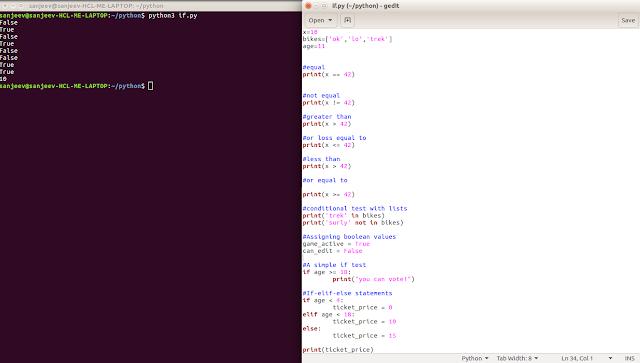Python If Statements - Supercoders | Web Development and Design | Tutorial for Java, PHP, HTML, Javascript Python If Statements - Supercoders | Web Development and Design | Tutorial for Java, PHP, HTML, Javascript

## Friday, November 30, 2018## Python If Statements

Introduction

If statements are used to test for particular conditions and respond approptiately.

```#conditional tests
x=10
bikes=['ok','lo','trek']
age=11

#equal
print(x == 42)

#not equal
print(x != 42)

#greater than
print(x > 42)

#or loss equal to
print(x <= 42)

#less than
print(x > 42)

#or equal to

print(x >= 42)

#conditional test with lists
print('trek' in bikes)
print('surly' not in bikes)

#Assigning boolean values
game_active = True
can_edit = False

#A simple if test
if age >= 18:
print("you can vote!")

#If-elif-else statements
if age < 4:
ticket_price = 0
elif age < 18:
ticket_price = 10
else:
ticket_price = 15

print(ticket_price)
```

###1.2.